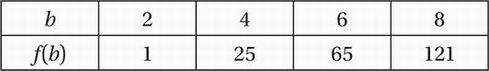# SAT Math Multiple Choice Question 654: Answer and Explanation

### Test Information

Question: 654

9.The table above shows ordered pairs that satisfy the function f. Which of the following could define f?

• A. f(b) = 3b2 - 2
• B. f(b) = 3b2 - 4
• C. f(b) = 2b2 - 7
• D. f(b) = 2b2

Explanation:

C

This question asks us to find the function that is satisfied by all four ordered pairs. Here, it is probably easiest to work by process of elimination, and cross off those choices that aren't satisfied by at least one of the ordered pairs. Let's consider the first ordered pair, (2, 1), and see which functions give an output of 1 for an input of 2:

(A) f(2) = 3(2)2 - 2 = 3(4) - 2 = 12 - 2 = 10

(No-eliminate.)

(B) f(2) = 3(2)2 - 4 = 3(4) - 4 = 12 - 4 = 8

(No-eliminate.)

(C) f(2) = 2(2)2 - 7 = 2(4) - 7 = 8 - 7 = 1

(Yes-don't eliminate.)

(D) f(2) = 2(2)2 = 2(4) = 8

(No-eliminate.)

So, it turns out we only have to test one ordered pair to get the right answer.